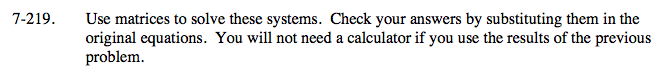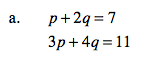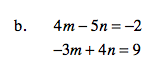Home > A2C > Chapter 7 > Lesson 7.3.5 > Problem7-219

7-219.
1. Use matrices to solve these systems. Check your answers by substituting them in the original equations. You will not need a calculator if you use the results of the previous problem. Homework Help ✎

1. p + 2q = 7
3p + 4q = 11

2. 4m − 5n = −2
−3m + 4n = 9First substitute matrices for the equations.

Multiply both sides by the inverse.

Solve for p and q.See part (a).